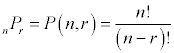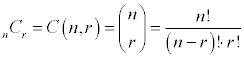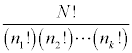# 7.6 - Counting Principles

Each branch of mathematics has its own fundamental theorem(s). If you check out fundamental in the dictionary, you will see that it relates to the foundation or the base or is elementary. Fundamental theorems are important foundations for the rest of the material to follow.

Here are some of the fundamental theorems or principles that occur in your text.

Fundamental Theorem of Arithmetic (pg 8)
Every integer greater than one is either prime or can be expressed as an unique product of prime numbers.
Fundamental Theorem of Algebra (pg 264)
Every polynomial in one variable of degree n>0 has at least one real or complex zero.
Fundamental Theorem of Linear Programming (pg 411)
If there is a solution to a linear programming problem, then it will occur at a corner point, or on a line segment between two corner points.

## Fundamental Counting Principle

If there are m ways to do one thing, and n ways to do another, then there are m*n ways of doing both.

The Fundamental Counting Principle is the guiding rule for finding the number of ways to accomplish two tasks.

### Examples using the counting principle:

Let's say that you want to flip a coin and roll a die. There are 2 ways that you can flip a coin and 6 ways that you can roll a die. There are then 2x6=12 ways that you can flip a coin and roll a die.

If you want to hit one note on a piano and play one string on a banjo, then there are 88 * 5 = 440 ways to do both.

If you want to draw 2 cards from a standard deck of 52 cards without replacing them, then there are 52 ways to draw the first and 51 ways to draw the second, so there are a total of 52*51 = 2652 ways to draw the two cards.

## Sample Spaces

A listing of all the possible outcomes is called the sample space and is denoted by the capital letter S.

The sample space for the experiments of flipping a coin and rolling a die are S = { H1, H2, H3, H4, H5, H6, T1, T2, T3, T4, T5, T6}. Sure enough, there are twelve possible ways. The fundamental counting principle allows us to figure out that there are twelve ways without having to list them all out.

## Permutations

A permutation is an arrangement of objects, without repetition, and order being important. Another definition of permutation is the number of such arrangements that are possible.Since a permutation is the number of ways you can arrange objects, it will always be a whole number. The denominator in the formula will always divide evenly into the numerator.

The n value is the total number of objects to chose from. The r is the number of objects your actually using.

The two key things to notice about permutations are that there is no repetition of objects allowed and that order is important.

### Examples of permutations:

Example 1: List all permutations of the letters ABCD

Now, if you didn't actually need a listing of all the permutations, you could use the formula for the number of permutations. There are 4 objects and you're taking 4 at a time. 4P4 = 4! / (4-4)! = 4! / 0! = 24 / 1 = 24.

This also gives us another definition of permutations. A permutation when you include all n objects is n!. That is, P(n,n) = n!

Example 2: List all three letter permutations of the letters in the word HAND

 HAN HNA HAD HDA HND HDN AHN ANH AHD ADH AND ADN NHD NDH NAH NHA NAD NDA DHA DAH DAN DNA DHN DNH

Now, if you didn't actually need a listing of all the permutations, you could use the formula for the number of permutations. There are 4 objects and you're taking 3 at a time. 4P3 = 4! / (4-3)! = 4! / 1! = 24 / 1 = 24.

### Finding Permutations by Hand

By hand, you can plug the values for n and r into the expression involving factorials and then simplify the ratio of the factorials as discussed in section 7.1.

However, there will always be n-r terms in common between the numerator and the denominator once the factorials are expanded. Those terms will divide out, leaving you with the first r terms of the expansion in the numerator. This gives us a shortcut for finding a permutation by hand.

nPr = first r factors of n!

### Finding Permutations with the Calculator

There is a permutation function on the calculator. On the TI-82 and TI-83, it is found under the Math menu, the Probability Submenu, and then choice 2. It is shown as nPr. Enter the value for n first, then the function, and finally the value for r.

## Combinations

Combinations were briefly introduced in section 7.5, but we will go into more detail on them here.

A combination is an arrangement of objects, without repetition, and order not being important. Another definition of combination is the number of such arrangements that are possible.The n and r in the formula stand for the total number of objects to choose from and the number of objects in the arrangement, respectively.

The key points to a combination are that there is no repetition of objects allowed and the order isn't important.

List all combinations of the letters ABCD in groups of 3.

There are only four combinations (ABC, ABD, ACD, and BCD). Listed below each of those combinations are the six permutations that are equivalent as combinations.

ABC ABD ACD BCD
ABC
ACB
BAC
BCA
CAB
CBA
ABD
BDA
DAB
DBA
ACD
CDA
DAC
DCA
BCD
BDC
CBD
CDB
DBC
DCB

We learned in the last section that combinations were symmetric. That is easy to see from the formula involving factorials. As an example, C(12,7) = C(12,5). Take whichever one is easier to find. Is it easier to find C(100,2) or C(100,98)? On the calculator it doesn't make much difference, by hand it does.

### Finding Combinations by Hand

By hand, you can plug the values for n and r into the expression involving factorials and then simplify the ratio of the factorials as discussed in section 7.1.

To simplify the ratio, you want the larger amount of terms to divide out. For example, if you need to find C(12,5), you could also find C(12,7). Either way, you're going to have a 12! in the numerator and both a 7! and 5! in the denominator. You would rather divide out 7! than 5!, because it leaves you less to work with. So, pick whichever r value is smaller, and then work with that combination.

nCr = (first r factors of n!) / (last r factors of n!)

It turns out the last r factors of n! is really just r!.

### Finding Combinations with the Calculator

There is a permutation function on the calculator. On the TI-82 and TI-83, it is found under the Math menu, the Probability Submenu, and then choice 3. It is shown as nCr. Enter the value for n first, then the function, and finally the value for r.

### Examples of Combinations

We experienced combinations with Pascal's Triangle, but there are other places they occur.

The old Illinois Lottery had 54 balls, of these 54 balls, six are chosen. None of the six can be repeated and the order of the six is not important. That makes it a combination: C(54,6) = 25,827,165.

I was told that on January 17, 1998, the Illinois Lottery will be changing to 48 balls, six of which are chosen. Now, the number of possibilities will be C(48,6) = 12,271,512

How many 5 card poker hands are there with 3 clubs and 2 diamonds? Well, there is no repetition of cards in a hand, and the order doesn't matter, so we have a combination again. Since there are 13 clubs and we want 3 of them, there are C(13,3) = 286 ways to get the 3 clubs. Since there are 13 diamonds and we want 2 of them, there are C(13,2) = 78 ways to get the 2 diamonds. Since we want them both to occur at the same time, we use the fundamental counting principle and multiply 286 and 78 together to get 22,308 possible hands.

### Difference between Permutations and Combinations

The distinguishing feature between Permutations and Combinations is not whether or not there is repetition. Neither one allows repetition. The difference between the two is whether or not order is important. If you have a problem where you can repeat objects, then you must use the Fundamental Counting Principle, you can't use Permutations or Combinations.

## Distinguishable Permutations

Consider all the permutations of the letters in the word BOB.

Since there are three letters, there should be 3! = 6 different permutations. Those permutations are BOB, BBO, OBB, OBB, BBO, and BOB. Now, while there are six permutations, some of them are indistinguishable from each other. If you look at the permutations that are distinguishable, you only have three BOB, OBB, and BBO.

To find the number of distinguishable permutations, take the total number of letters factorial divide by the frequency of each letter factorial.where n1 + n2 + ... + nk = N

Basically, the little n's are the frequencies of each different (distinguishable) letter. Big N is the total number of letters.

### Example of distinguishable permutations

Find the number of distinguishable permutations of the letters in the word MISSISSIPPI

Here are the frequencies of the letters. M=1, I=4, S=4, P=2 for a total of 11 letters. Be sure you put parentheses around the denominator so that you end up dividing by each of the factorials.

11! / ( 1! * 4! * 4! * 2! ) = 11! / ( 1 * 24 * 24 * 2 ) = 34,650.

You may want to do some simplification by hand first. When you simplify that ratio of factorials, you get that there are 34,650 distinguishable permutations in the word MISSISSIPPI. I don't want to list them out, but it's better than listing out all 39,916,800 permutations of the 11 letters in MISSISSIPPI.